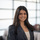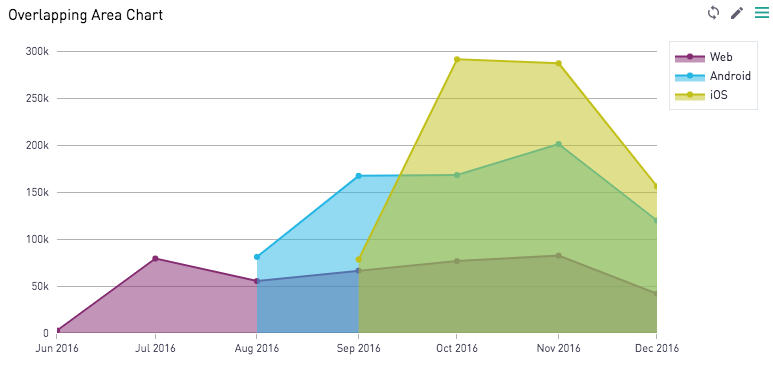# Overlapping Area Chart — Plot.lyWe can create overlapping area charts in Periscope with the Plot.ly integration! These offer a better way to see the exact value per shaded region than stacked area graphs. If your purpose, however, is to draw attention to the total value across all shaded a regions, a stacked area graph may be better suited.

The Python 3.6 code used to generate the above area chart can be found below. The data shows the number of users who joined a fictional gaming site, segmented by platform. Our SQL output returns the following 3 columns:

• dte: the month the users joined
• platform: the platform the users are active on
• count: the number of users per dte/platform

Code is inspired from Plot.ly's documentation. Take a look there for further customizations you can do with plot.ly area charts!

```# SQL output is imported as a dataframe variable called 'df'
import pandas as pd
import plotly.plotly as py
import plotly.graph_objs as go

# Our SQL output has 3 columns. One with the date (dte), one with the platform, and one with the count of users in each dte/platform combination.
# For use in plot.ly, we must pivot the table such that each platform is its own column. If your data is already pivoted, you can avoid this step
df=pd.pivot_table(df,index='dte',columns='platform',values='count')
df=df.reset_index()

# Build Graph
web = go.Scatter(
x=df['dte'],
y=df['web'],
fill='tozeroy',
line=dict(
color='rgb(145, 50, 125)',
),
name = 'Web'
)
android = go.Scatter(
x=df['dte'],
y=df['android'],
fill='tozeroy',
line=dict(
color='rgb(43, 190, 231)',
),
name = 'Android'
)
ios = go.Scatter(
x=df['dte'],
y=df['iOS'],
fill='tozeroy',
line=dict(
color='rgb(200, 200, 25)',
)  ,
name = 'iOS'
)

data = [web, android, ios]

layout = go.Layout(
margin=dict(
l=50,
r=50,
b=50,
t=10
)
)
fig = go.Figure(data=data, layout=layout)

# Use Periscope to visualize a dataframe, text, or an image by passing data to periscope.table(), periscope.text(), or periscope.image() respectively.
periscope.plotly(fig)
```